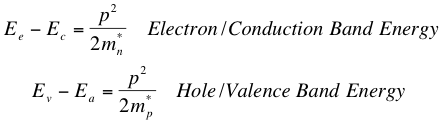# 2. Direct Semiconductors

## The Dynamics of Charge Carriers

To discuss the different types of absorption in semiconductors, let’s understand just a little bit about the dynamics of charge carriers, and the relationship between photon energies, the band gap in semiconductors, and momentum of charge carriers. The force on a charge carrier in a crystal is F = m*a, or the product of “effective mass” and acceleration. This makes the kinetic energy of a charge carrier in a crystalwhere p is the momentum of the charge carrier and m* is the effective mass. Furthermore, during electron excitation, a photon is absorbed by an electron, and therefore the energy difference between the electron’s final and initial energy states is the energy of the photon. Thus we have thatwhere Ee is the original energy of the crystal, Ea is the final state of the crystal after the photon is absorbed, h is Planck’s constant, and f is the corresponding frequency of the photon.

The matching equations for the energies of an electron in the conduction band and hole in the valence band are as follows1:where Ec is the energy level of the minimum edge of the conduction band, Ev is the energy at the edge of the valence band, and mn* and mp* are the effective masses of electrons and holes respectively (which are actually different from each other). These equations mean that the electron’s energy due to its momentum is the difference between its “relaxed” state of the crystal before photon absorption and the conduction band energy level, and the hole’s energy is the difference between its energy state in the valence band and the energy of the crystal after photon absorption. These equations are just a result of conservation of energy and momentum; the photon absorption must result in an energy change in both the electron and hole in such a way that the momentum of each is increased with kinetic energy. The momentum imparted on the crystal is small, but the quantity is squared for a greater effect.Fig. 1: The energy level difference, Eg, between the edges of the conduction and valence bands constitutes the minimum energy a electron requires from a photon to become conductive (a free charge carrier).

## Absorption Coefficient for Direct Semiconductors

Adding the two previous equations together produces a very useful result for explaining absorption in a certain class of semiconductors. If we do this, we getwhere Eg (illustrated in the Fig. 1 above) is the energy required to cross the band gap and hf is the energy of the absorbed photon1. It turns out this equation holds true for a type of semiconductor called a direct semiconductor, because the absorption conditions are purely based on the band gap of the material and the photon energy. This is because the curves relating energy to crystal momentum are aligned, and therefore the crystal momentum increases directly as a result of increased photon energy.Fig. 2: The valence band and conduction band curves in a graph of energy vs. crystal momentum are aligned if the semiconductor has a direct band gap.

Source: Wikipedia Commons.

The next question to ask is: what does this energy-momentum relationship have to do with absorption probability? According to our relation, the crystal momentum increases with energy; however, we know that absorption must depend on the density of electrons and holes in the material. It is known that the density of electrons increases (see Fermi Levels) downward in energy from the valence band edge, while the density of holes increases upward in energy from the conduction band edge. Therefore, the absorption coefficient previously discussed increases with increasing photon energy above Eg. Using our above equation relating the band gap and photon energies and a theoretical treatment (not discussed here), we obtain the expression for the absorption coefficient of a direct semiconductor2:Thus, the absorption of a semiconductor is dependent on the frequency (or wavelength) of the photon and the band gap, and the constant C is approximately 2 x 104 for all direct semiconductors if α is expressed in cm-1 and hf and Eg in eV (electron-Volts).

## Generalizing the Absorption Coefficient

To generalize mathematically the energy relations given in the first section of this page for the valence and conduction bands, we can think of the curves of the two bands as being “misaligned”, with minimums/maximums being separated by some distance on the crystal momentum axis. Therefore, the minimum of the conduction band would be at some finite value of crystal momentum2, such thatwhere p0 and p0' are the respective shifts in the crystal momentum for the conduction and valence bands (see Fig. 3 below).

We can see from these equations that if p0 = p0', the semiconductor has a direct band gap, because an increase in photon energy directly correlates to an increase in crystal momentum. If p0p0', then the band gap is called indirect. We will discuss this in the next section, and this will include the more complicated expressions for the absorption coefficient of indirect semiconductors.Fig. 3: The indirect band gap is shown here, and one can see how the minimum energy (y-axis) of the indirect band gap has an offset crystal momentum (x-axis) from the valence curve.

Source: “An Introduction to Solar Cell Technology”. <http://cnx.org/content/m41217/latest/>

## References

1. Goetzberger, Adolf et.al. Crystalline Silicon Solar Cells. Chichester: John Wiley & Sons Ltd., 1998.

2. Green, Martin A. Solar Cells: Operating Principles, Technology, and System Applications. Englewood Cliffs: Prentice-Hall, Inc., 1982.Full book ordering information at www.pv.unsw.edu.au.